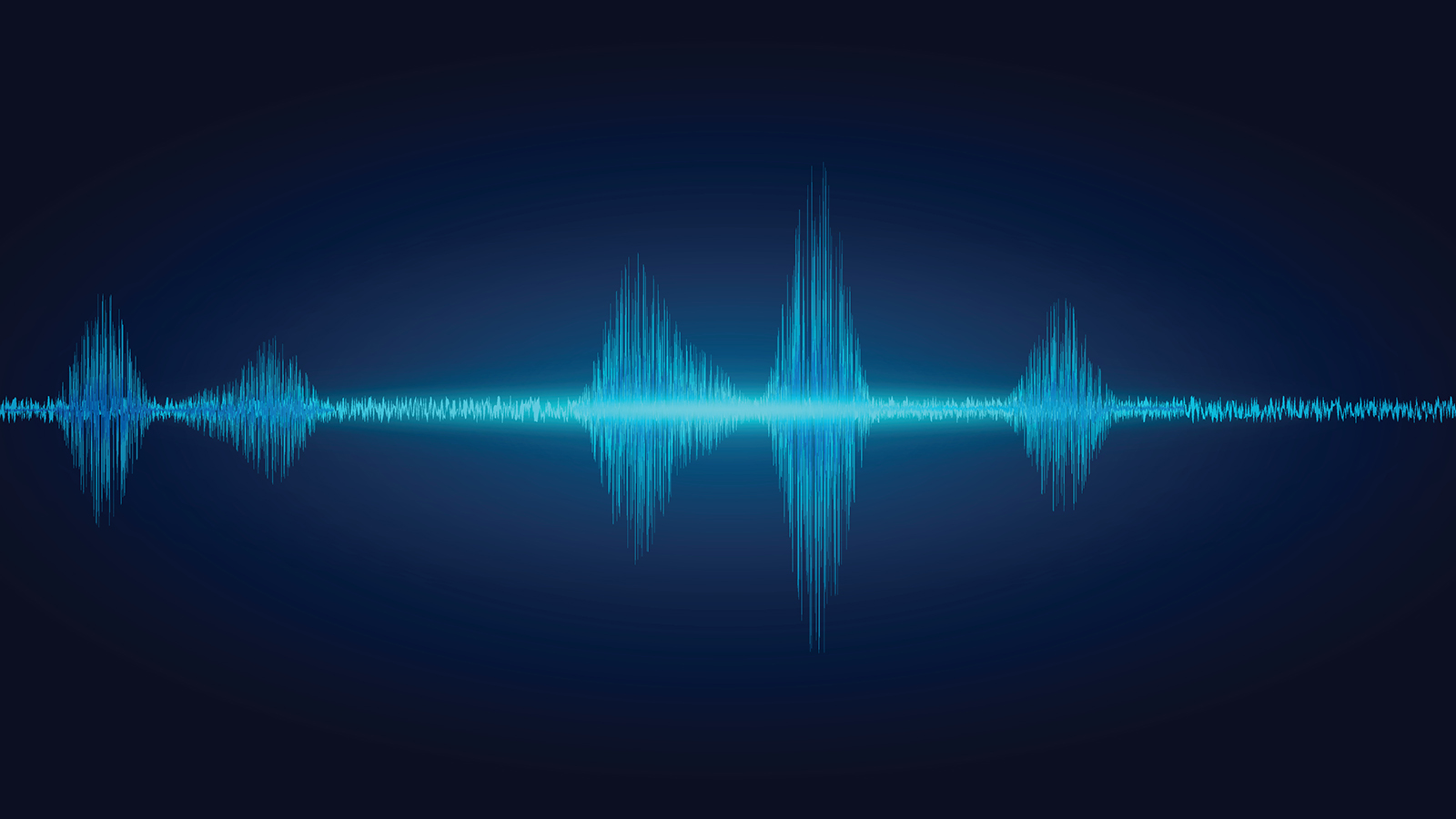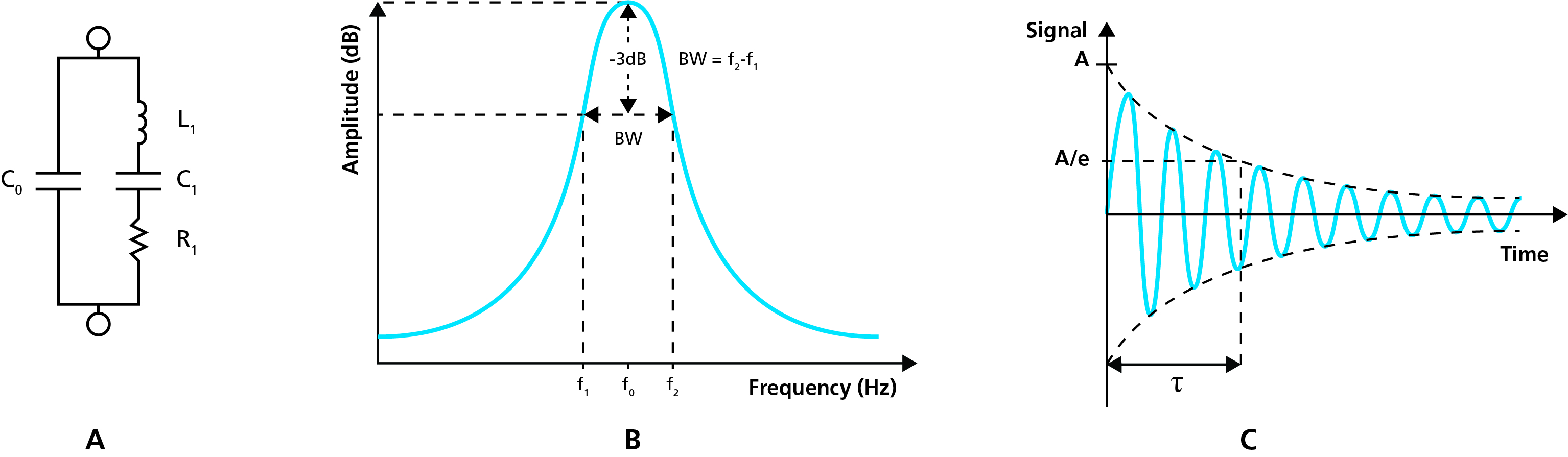Malin Edvardsson Sep 22, ’20 < 5 min

# Different ways of measuring the Dissipation

## Surface Science Blog

Extended QCM:s, such as for example QCM-D, QCM-A, and QCM-I, all provide information about the energy loss in the system under study. However, there are several ways of measuring these energy losses and not all QCM:s collect the information in the same way. Here we tell you more about the different approaches and their respective pros and cons.

## Why should you measure the dissipation?

As discussed in previously published posts, the dissipation contains information about the material under study. This is particularly apparent when you study viscoelastic layers, where the wave propagation is very different from that of quartz. The energy losses in such films can be significant. Information about the dissipation is therefore very valuable, not only to determine what approach to use for the mass and thickness quantifications, but also to be used as input for the viscoelastic modelling, when applicable .

## Different ways to measure the Dissipation

There are three main ways to extract information about the energy loss, the D-value, in the system. The three ways are:

1. impedance spectroscopy
2. the decay time of the oscillation
3. the resistance

### 1) Impedance and admittance measurements

One way to assess the D-value is to use impedance or admittance spectroscopy [2, 3]. A sinusoidal voltage, V, is applied over the crystal and the resulting current, I, is measured as a function of the frequency of the applied voltage. The ratio of the voltage and current will then give the frequency-dependent impedance, Z. The admittance, Y, which is the reciprocal of the impedance, can also be extracted. This result can then be used to quantify the parameters of the electrical equivalent circuit, Fig. 1A, of which L1 and R1 are needed to calculate D.

The D value can also be obtained by measuring the bandwidth, or half band­width at half maximum, Γ, of the reso­nance peak, Fig. 1B, and then using Eq. 1.Figure 1. A) Equivalent circuit of a quartz crystal sandwiched between two electrodes, the motional arm being the branch to the right. B) schematic illustration of the resonance peak, showing the center frequency, f0, and the bandwidth, BW, which gives the D-value. C) A damped oscillation with maximum amplitude A and decay time τ.

### 2) Decay time measurement, pinging

Another approach to extract the D is to measure the decay time, τ, of the crystal oscillation, Fig. 1C, and use Eq. 2 [4-5]. In this so-called pinging approach, the crystal is rapidly excited to resonance, the driving voltage is then turned off and the decay time of the oscillation is monitored. D can then be extracted along with the resonant frequency of the sensor.### 3) Resistance measurements

The resistance, R, is essentially equivalent to the resistor in the motional arm in the circuit in Fig. 1A and can be measured, for example, via an advanced oscillator circuit .

## How do the different methods to measure the Dissipation compare?

There are three main ways of measuring the energy losses in the system and to get the D-value, impedance-, pinging-, and resistance measurement. Each method is associated with some pros and cons such as time resolution and information content. Download to the overview to learn more about the appproaches and what the respective pros and cons are.## References

1. V. Voinova et al.; Physica Scripta, 59: 391-396, 1999
2. A. Buttry and M. D. Ward; Chemical reviews; 92 (6): 1355-1379, 1992
3. Wegener et. Al, Cell Biochemistry and Biophysics, 34 (1): 121-151, 2001
4. Rodahl, et al., Review of Scientific Instruments, 66 (7): 3924-3930, 1995
5. Rodahl, et al., Review of Scientific Instruments, 67 (9): 3238-3241, 1996
6. J. Martin, et al.; Sensors and Actuators A; 44: 209-218, 1994

## Explore the blog

You have only scratched the surface.